## Data Structures And Algorithms

The data structures and algorithms  is the one of the  important topic for computer science students. In computer programming , the data structure  is a fundamental building block   for  developing an efficient software application .

And therefore , the programmer must have a good knowledge and understanding of  the data structures.

The Data Structure defines the  way in which various  program data elements are organized and stored into the memory  so that the data can be used efficiently.

This Data Structures And  Algorithms tutorial extensively covers  all the important  topics  such as types Of Data structures , Linear And Non-Liner Data structures , Array, Pointer, Structure, Linked List, Stack, Queue, Graph .

The data operations such as  Traversing , Searching , Sorting And other topics related to the algorithms.

## What Is Data Structure ?

The data structure  is the most fundamental building block  in  computer science . And  therefore ,  a  good knowledge of  data structure  is  important  for  a  software  application  design and  development .

The  data structure  mainly  deals with  the organization  of  the data  in a program  and  the operations  allowed  on that data .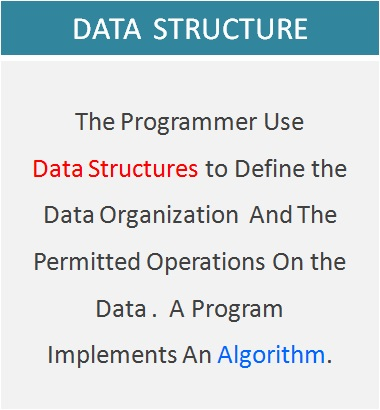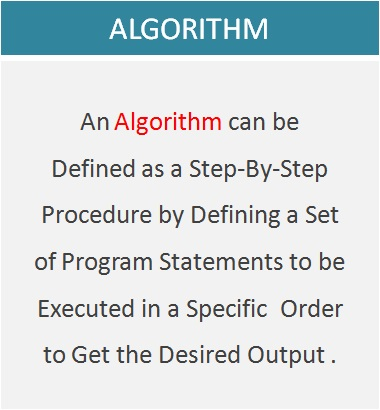In computer science , the data structure is a specific way to organize and store  the data in a computer’s memory. The programmer can organize the data and also define the permitted operations on the data with the help of data structure so that the data can be  used  more  efficiently .

In most simple terms , anything  that used  to store and organize the program data , while writing a program code is a  data structure .

Some  data structures  and the operations allowed are  predefined in the programming  language itself .Whereas the programmer can also  define  a  data structure  to meet any specific  requirement . Such DS are referred as  user defined  data structures.

## What Is the Use Of Data Structure ?

The  computer programs  are  designed  to handle different kinds  of  data  such as text , numbers , images  , audio , video  and  other types .

The  software  programs  are  designed  and developed for different types of applications.  And  therefore , the computer programmers  have  to deal with all kinds of data   such  as  text  and  numerical  data , images , audio  and  video  .

From  the software application performance point of view  , the efficiency and the performance of the software program depends upon  how  the data is stored , organized  and  grouped  together, during the  program execution . And therefore computer scientist , software engineers , software developers must have a good knowledge of  data structures .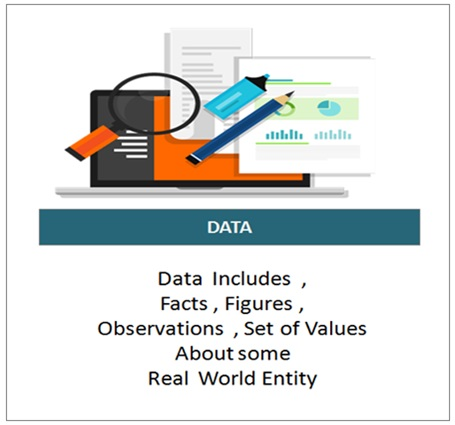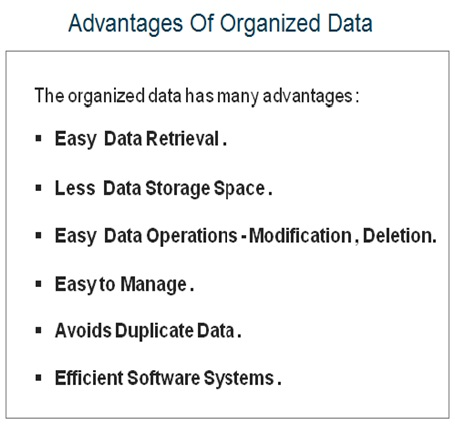## Types Of Data Structures

The data structures  ( DS ) can  be  broadly  grouped  in to two basic  types and that  includes Primitive Data Structures    and Non-Primitive  Data Structures  . These  DS  are  grouped  on the basis  of  either  pre-defined  in the programming language  or  defined by the user   .

Some  data structures  are  built  in to the  programming  language  itself  which are readily  available  to the  programmer for  its  use  and these DS  are referred  as  primitive  data structures  .

For  example  , the most  common  primitive  data structures  which  are  generally supported by  almost  all  programming languages  include  Integer  ,  Float  , Character  and Boolean  .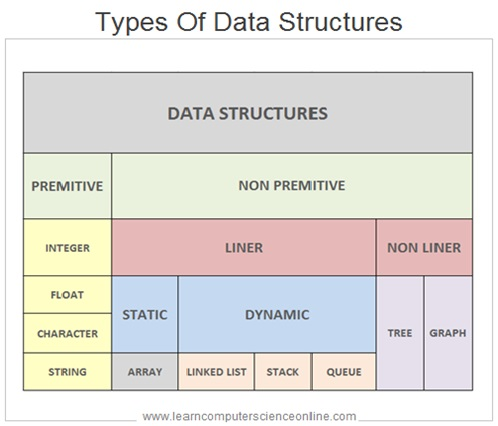The  Non-Primitive  Data Structures  can be further classified  as  Linear  DS  And  Non Linear DS . The  Linear  DS can be further  Classified  in  two further subcategories  such as  Static and  Dynamic  depending upon the ability of the DS to expand  or shrink  as per the program needs .

The Static Linear  DS includes Arrays , whereas  the  Dynamic  linear  DS  includes Linked List  ,  Stack  and Queue.  The  Non Linear DS  includes  Trees  and  Graph . Let us  now discuss  each  of these DS  one by one in detail .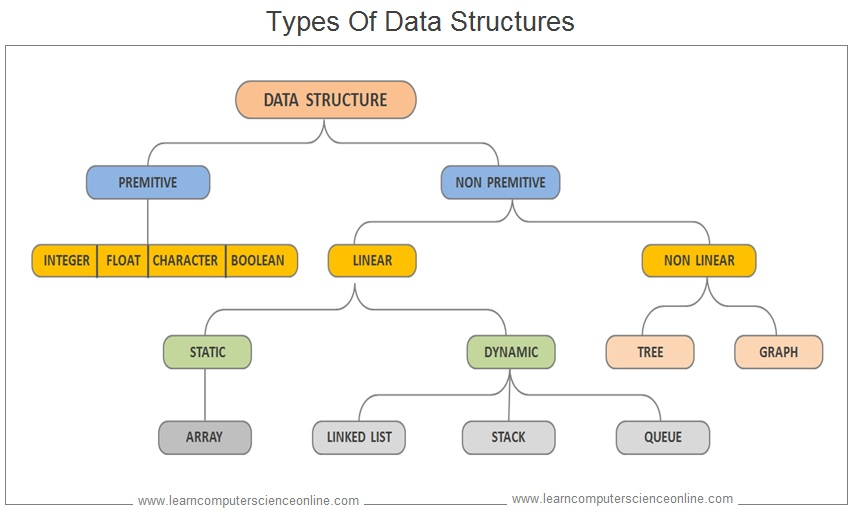## Primitive Data Structures

The primitive data structures are  the programming language defined data structures which are built into the programming language itself , which programmers can readily use in the program . Almost  all programming  languages  support the primitive  data structures  .

The examples of primitive data types include integers , floating point numbers and one single individual characters in text.

The primitive data types are readily available to the programmer and  doesn’t require a large amount of data for representation.

Each Character correspond to a single specific reference value  in an ASCII table. The Integers numbers can represent a small to a very large values without the decimal point.

Whereas the Boolean values needs only two possible binary numbers that is either 0 ( zero ) or 1 ( one ).

## Primitive Data Structure Types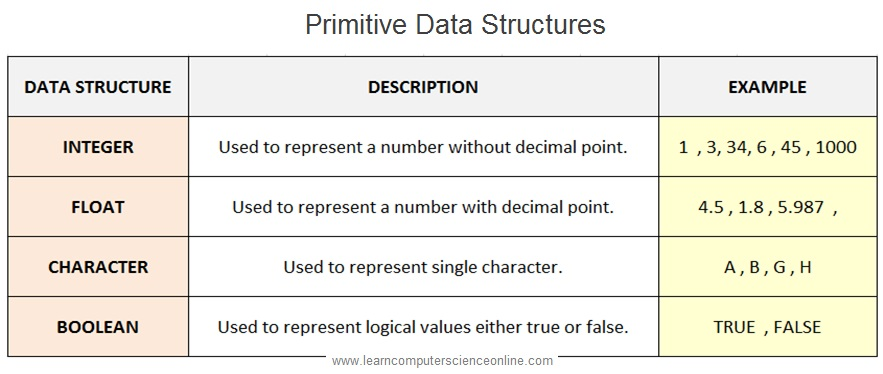## Non-Primitive Data Structures

The non-primitive data structures are  user ( programmer ) defined data structures which are  also  referred  as user  defined  data structures .

The  non-primitive data structures are  derived  from  primitive  data types  by combining  two  or  more  primitive  data  structures. These DS can be further  sub divided  as  linear  and  non- linear  data structures .

The Non-primitive data types are not pre-defined in the programming language . And therefore , the programmer has to define as per the program requirements.

## Characteristics Of Non-Primitive Data StructuresFor example , In  Java programming language, the non-primitive data types are referred as  “objects” because these objects are  created run-time by defining Classes . An object may contain any primitive type of data as per the program requirements.

### Arrays

In computer science  , an  array  is a linear data structure  ( also commonly referred as an array  )  is  collection  of  elements  of  same  data type . An  array  is  linear data  structure  because  the array  elements  (  Values  )  are traversed  in sequential  manner .

The simplest type of linear data structure is a one-dimensional  array . An array   is organized  in a single  row  consisting  of  number of pre-defined number of data elements . These data elements are stored in a contiguous memory locations allocated by the operating system.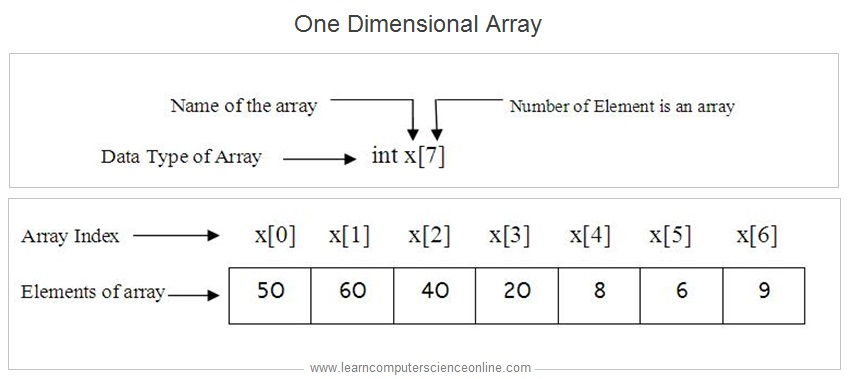### Where To Use Arrays ?

While writing a programming code , sometimes the  programmer  is required  to store  large number  values of the  same data type  such as  student marks , employee contact numbers , customer order numbers and so on .

In such situations , one solution is to declare large number of variables using different variable names which is not a practical solution .

Instead of creating large number of variables , the programmer can  use an  array  DS , which  allows the programmer ,  to store large  number of values  of the  same data type    using   same  variable name .

Let us consider one simple example of designing a software for a school . If a programmer wants to store the names of  100 students , then programmer has two options .

In the first option ( Not recommended ) , a programmer can crate 100 variables of string type and assign student name to each of these variable . This program will work but it is the most inefficient program .

In the second option ( Recommended ) , the programmer can simply create an array of students name ( String data type ) with capacity to store 100 names . This is a much better way of organizing program data .

### Types Of Arrays

Depending upon the programming needs , the programmer can choose different types of arrays which include one , two and multi-dimensional arrays.

### Examples Of One , Two , Three Dimensional Arrays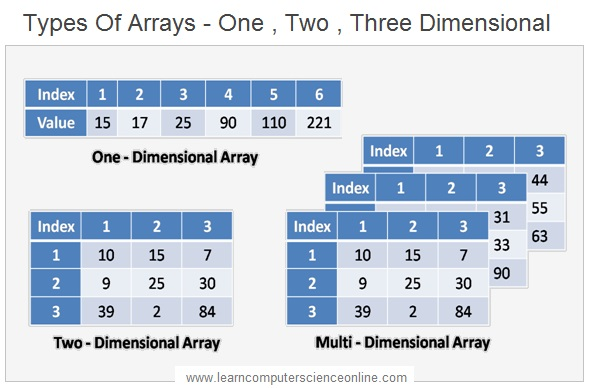### Linear Dynamic Data Structures

In computer science , the linked list is a linear dynamic data structure. It is a collection of data elements called nodes . Each node consist of two fields . The first field of the node contains the actual value whereas the send field of the node contains a pointer ( a memory address ) which  points  to the next node .

In simple terms , the linked list  can be visualized as a chain of nodes  , where every node points  to  the  next node. And therefore it offers advantage of flexibility of size.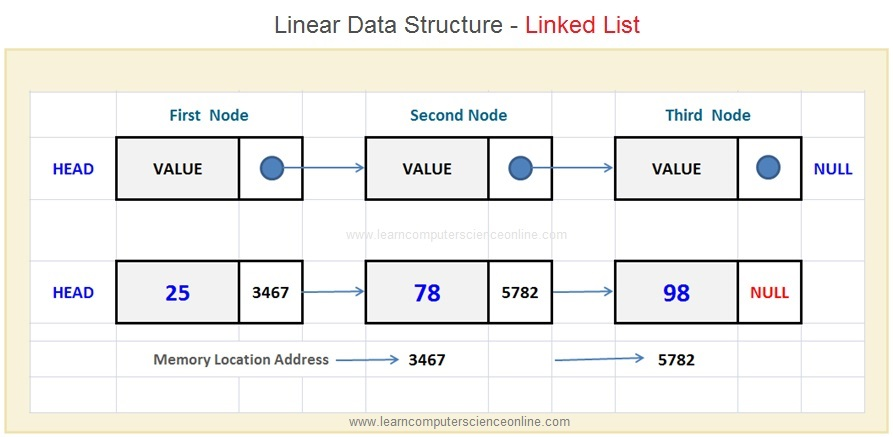The linked list is a dynamic linear data structure.  That  means  ,  the  programmer can dynamically  modify  the  size  of the  linked list  during the program run-time  as per the needs .

This  was not  possible  in case  of   array   which  is  static  data  structure  and programmer has to define the  .

The linked list  does not have indexing  system  to access  specific data element  and makes use of traversing  to perform desired operation .

### Linear Dynamic Data Structures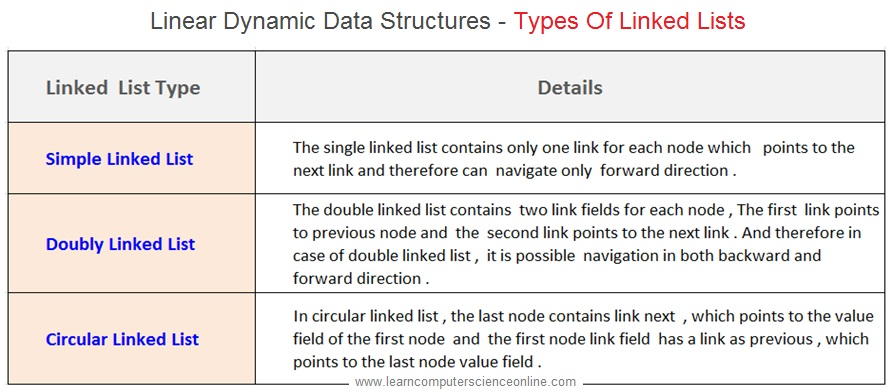### Trees And Graphs

A non-linear data structure is a data structure in which a data item is connected to several other data items and the data items are not organized sequentially. And therefore it  is called as non-linear data structure.

In case of non-liner data structure it is possible for a data item to be connected with one or more data items. The examples of non-linear data-structures includes  Graphs and Trees.### Abstract Data Type ( ADT )

Abstract Data Type ( ADT ) is an important topic in computer science . In order to understand the concept of ADT  , we need to familiar with some fundamental programming concepts  such as  program  variables , different  data types  ,  OOP  concepts  such  abstraction  , encapsulation  and the concept of class .

While writing a program code , the  programmer  needs  to  declare  the variables  to store the data . This program data  will be operated upon as per  the program instructions  .  The  variables are named  memory locations  where different  types of data is stored  that can be referred in program  code  with different variable names .

The  program data can be of different types  such as integer , float , string , Boolean . The data type for a variable  signifies  the  type of the data that will be stored  and  the associated  operations .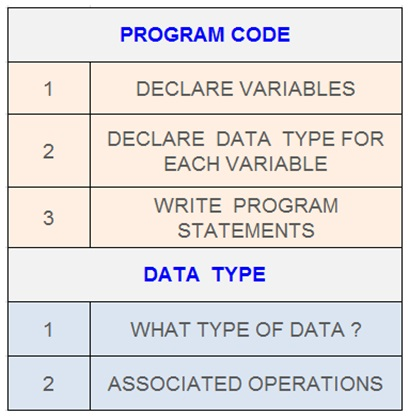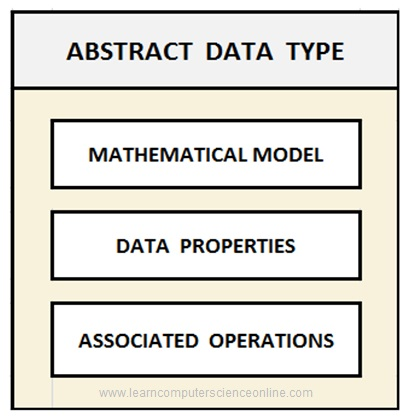An  ADT – abstract data type represents a mathematical model  which provides an abstracted view of the data structure in terms data properties  and the  operations allowed on the data .

Each programming language provides some predefined data types  in built in to the language which are referred as primitive data types . In some situations the primitive data types  may not be sufficient  and  in such cases the programmer can define  a data type  along with its associated  operations .

### Abstract Data Type ( ADT ) Examples

In  Object Oriented Programming ( OOP )  the concept of  Class  represents  an   ADT  .  We can define  a Class   as  a  blueprint for  creating   the  objects  .

In OOP  , the Class consist of data members and  the associated operations which operates on the data members  . The Class  is an example of  an ADT  which binds together  the  data  and  the  associated operations .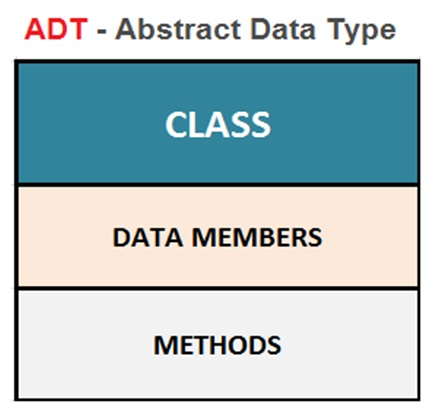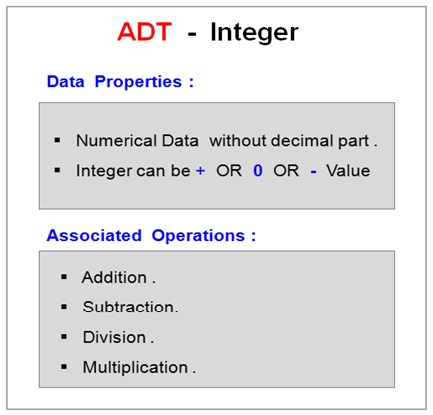Let  us  consider one example of  an  integer data type is one of the most commonly used primitive data type .

The mathematical concept of an integer, along with operations that manipulate integers, form a ADT. The int variable type is a physical representation of the abstract integer.

The int variable type is an ADT because it has some well  defined  data properties  and  the operations that are associated  with integer such as addition , subtraction   and other  operations .

## Data Structures And Algorithms

### Introduction To Algorithms

The  computer program  is  a set  of program instructions  which  provides the direction to the computer’s  CPU  to execute the desired  operations  as per the program  instructions .

Before writing a computer program code , the programmer has to first decide the logic of the program and then translate this logic in to the sequence of logical steps  which implements that logic  to solve  the problem  in a particular manner  and this logic  is referred as an program algorithm .

### What Is Algorithms ?There can be many possible solution to a problem .  And therefore , the programmers must logically think  and explore different  algorithms on its merits.

The programmer must explore the various alternate approaches to find  the best possible algorithm that provides the solution  which is most efficient .

Each computer program implements a specific algorithm . The  Efficiency  of  the  computer system  to solve a particular problem depends upon  the  efficiency  of the program code  which  directs the computer to solve that problem  in a particular manner .

Now  we are going to discuss   various  aspects of  algorithm in detail .

### What Is Algorithms ?

An Algorithm can be defined as a step-by-step procedure by defining a set of instructions which implements a specific logic and  executed in a specific  order to get the desired output .

Each Algorithm only provides one specific  approach which leads to the solution. An algorithm only indicates an approach that leads to one solution .

And therefore, algorithms are programming language independent and can be  implemented using  any  programming language.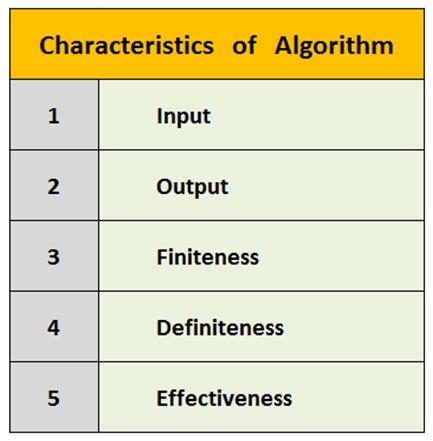The  computer programmers create algorithms during the software design stage  before  writing  a  program code  . Each  algorithm provides different approach to solve the problem in specific way .

The programmers  then analyze each of these algorithms against some  standard evaluation criterion. These algorithm are evaluation on two criterion that includes  Time Complexity  and Space Complexity to evaluate the algorithm performance .

After careful evaluation , the programmers then   finally  select  the algorithm for its implementation in the program code .

The algorithm which provides the best  approach  to solve a particular problem is implemented in the final code in the software project .

## Data Structures And Algorithms

### Classification Of Algorithms

There are many types of  Algorithms that can be classified depending upon many parameters . However some of the fundamental types of algorithms include :

Join The Best Seller

## Learn Computer Science And Programming Fundamentals

This is the most comprehensive  and unique  Computer Science  And Programming Fundamentals course Online which will give you in depth understanding of most important fundamental concepts in computer science And Programming .

Don`t copy text!